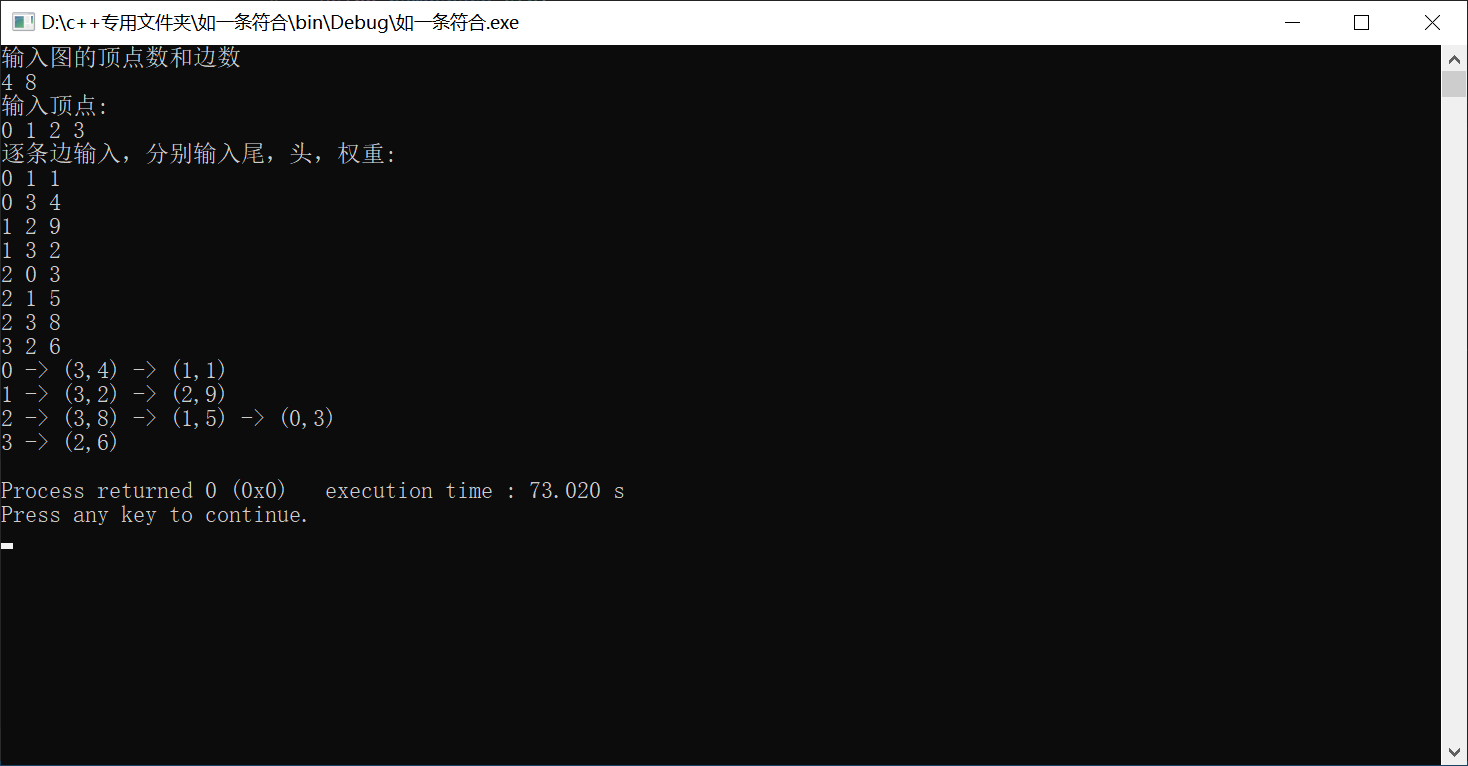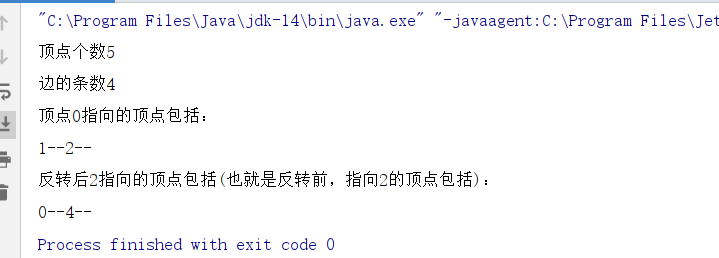• 节点内容只有一整型标识符ID，可以更清楚写typedef int ID 邻接表采用链表数组，头插法 耐人寻味的细节 邻接表在java里这样写Bag<Integer>[] adj,我想c++至少这几种方法： 一、固定大小的数组list<...
Graph API必要的说明

算法第四版 人民邮电出版社，原书是java实现
节点内容只有一个整型数标识符ID，可以更清楚写typedef int ID
邻接表采用链表数组，头插法

耐人寻味的细节

邻接表在java里这样写Bag<Integer>[] adj,我想c++至少有这几种方法：
一、固定大小的数组list<int> adj[MAX_V];
二、灵活的数组vector<list<int>> adj;
三、指针，内存在堆vector<list<int>>* adj; vector<list<int>*> adj; vector<list<int>*>* adj;
哪个好呢？这里用了和原文不同的写法，用了第二种，但adj.emplace_back(*new list<int>());虽然链表还是在堆上开辟，但vector实体在函数栈内。
严格说，和原文Bag<Integer>[] adj等价的写法是vector<list<int>*>* adj;图类的实例只保存一个指针，所有数据都在堆上申请，调用时需要用的指针或者解引，像adj->at(i)或(*adj)[i]有点麻烦。
已修改，typedef list<int>* ListPtr;	vector<ListPtr>* m_adj。
测试实例如左图，开头两行是节点数和边数。当构造函数再调用其他构造函数
JAVA是可以的，但是，C++似乎不行，所以，用一个私有函数完成构造函数的活儿。
package source;

public class Hero {
private int hp;
private double damage;
private String name;

Hero(String name){
this.name = name;
}

Hero(String name,int hp,double damage){
this(name);//调用第一个构造函数
this.hp = hp;
this.damage = damage;
}
}

Graph.h
#pragma once
#include<string>
#include<fstream>
#include<iostream>
#include<list>
#include<vector>
using namespace std;

#define out(x) cout<<x<<" "
#define hh printf_s("\n")
#define random(x) rand()%(x)

typedef  list<int>* ListPtr;

/*********************如无必要，勿增实体************************/
class Graph
{
public:
Graph(int V);
Graph(string file);//从文件读入图数据

int V();
int E();

void addEdge(int v, int w);//向图中添加边v-w,顶点已存在
list<int> adj(int v); // 和v相邻的所有顶点
string toString();//对象的字符串表示

private:
int m_V=0;//节点数
int m_E=0;//边数
vector<ListPtr>* m_adj=nullptr;//邻接表

void initGraph(int V);//创建一个有V个顶点但不含有边的图
string intToStr(int n);
bool isParallel_edges_self_loop(int v,int w);//检测平行边和自环
};

void testG();
void setGraphTxt(int V,int E);//随机生成图的构造文件graph.txt

Graph.cpp
#include "Graph.h"

Graph::Graph(int V)
{
m_V = V;
m_E = 0;

m_adj = new vector<ListPtr>(V, nullptr);
for (int i = 0; i < V; i++)
{
m_adj->at(i) = new list<int>(0);
}
}

Graph::Graph(string file)
{
ifstream in(file, ios::in);
if (!in) {
printf_s("file open error.");
return;
}

in >> m_V;

initGraph(m_V);

int E = 0;
in >> E;//不一定等于最后的边数，因为可能不包含自环和平行边
int w, v;
for (int i = 0; i < E; i++)
{
in >> w >> v;
addEdge(w, v);
}
in.close();
}

int Graph::V()
{
return m_V;
}

int Graph::E()
{
return m_E;
}

void Graph::addEdge(int v, int w)
{
if (isParallel_edges_self_loop(v, w)) return;
m_adj->at(v)->push_front(w);
m_adj->at(w)->push_front(v);
++m_E;
}

list<int> Graph::adj(int v)
{
ListPtr p = m_adj->at(v);
return *(p);
}

string Graph::toString()
{

string s = intToStr(m_V) + " vertices, " +intToStr(m_E) + " edges\n";

for (int i = 0; i < m_V; i++)
{
s += intToStr(i) + ": ";
for(auto adj:*(m_adj->at(i)))
{
s += intToStr(adj) + " ";
}
s += "\n";
}
return s;
}

void
inline Graph::initGraph(int V)
{
m_V = V;
m_E = 0;

m_adj = new vector<ListPtr>(V, nullptr);
for (int i = 0; i < V; i++)
{
m_adj->at(i)= new list<int>(0);
}
}

string
inline Graph::intToStr(int n)
{
char int_s = { 0 };//末尾加上'\0'
_itoa_s(n, int_s, 10);// 10 表示十进制
return string(int_s);
}

bool Graph::isParallel_edges_self_loop(int v,int w)
{
if (v == w) return true;
for (auto& n : *(m_adj->at(v))) {
if (n == w) {
return true;
}
}
return false;
}

void GFile(int V, int E)
{//指定节点边数生成随机图
ofstream write("graph.txt");
if (!write) {
out("file opens error !"), hh;
return;
}
write << V << endl;
write << E << endl;
srand((unsigned)time(0));
for (int i = 0; i < E; ++i) {
int from = random(V);
int to = random(V);
write << from << " " << to << endl;
}
write.close();
}

void testG()
{
GFile(19, 135);
Graph G("graph.txt");
cout << (G.toString()) << endl;
}


data.txt
13
13
0 1
0 2
0 5
0 6
5 3
5 4
3 4
4 6
7 8
9 10
9 11
9 12
11 12

补充

注意对非法数据的处理，边数和顶点数非负

节点i的度数，m_adj->at(i)->size()


展开全文数据结构 链表 c++
• 有向图邻接表的创建与输出 算法： #include <iostream> using namespace std; #define MaxVexNum 100//顶点的最大个数 typedef char VerTexData;//顶点数据类型 typedef int EdgeData;//权值的类型 ...
有向图的邻接表的创建与输出
算法：
#include <iostream>
using namespace std;
#define MaxVexNum 100//顶点的最大个数
typedef char VerTexData;//顶点数据类型
typedef int EdgeData;//边权值的类型
typedef struct node//边节点
{
int dest;//目标节点位置
EdgeData cost;//边的权值
struct node *link;//下一边链接指针
}EdgeNode;
typedef struct//顶点结点
{
VerTexData data;//顶点数据域
EdgeNode *adj;//边链表头指针
}VerTexNode;
typedef struct//图的邻接表
{
VerTexNode VexList[MaxVexNum];//邻接表
int n,e;//图中当前的顶点个数与边数
}AdjGraph;
void CreatGraph(AdjGraph &G)//创建邻接表
{
int tail,head,weight;
cout<<"输入图的顶点数和边数"<<endl;
cin>>G.n>>G.e;
cout<<"输入顶点:\n";
for(int i=0;i<G.n;i++)//输入顶点信息
{
cin>>G.VexList[i].data;
G.VexList[i].adj=NULL;
}
cout<<"逐条边输入，分别输入尾，头，权重:\n";
for(int i=0;i<G.e;i++)
{
cin>>tail>>head>>weight;//逐条边输入
EdgeNode *p=new EdgeNode;
p->dest=head;p->cost=weight;
p->link=G.VexList[tail].adj;
G.VexList[tail].adj=p;
}
}
void ShowGraph(AdjGraph G)//输出邻接表
{
for(int i=0;i<G.n;i++)
{
cout<<G.VexList[i].data;
EdgeNode *p=G.VexList[i].adj;
while(p!=NULL)
{
cout<<" -> ("<<p->dest<<","<<p->cost<<")";
p=p->link;
}
cout<<"\n";
}
}
int main()
{
AdjGraph G;
CreatGraph(G);
ShowGraph(G);
return 0;
}

输出结果:展开全文c++ 数据结构 算法
• 图的表示（建立）有两种方法： ①邻接矩阵：A(i,j)=1表示i,j存在一条，空间复杂度O(n^2)，稠密图 ...有向图 注意理解头插入节点的过程 int n,m;//n表示城镇个数，m表示道路条数 struct LinkNode//列表
图的表示（建立）有两种方法：
①邻接矩阵：A(i,j)=1表示i,j存在一条边，空间复杂度O(n^2)，稠密图
②邻接表：只记录存在的边，Vector+List的数据结构，稀疏图

邻接矩阵的图建立这里不做赘述，接下来我们看一下邻接表的图建立：

<1>有向图
注意理解头插入节点的过程
int  n,m;//n表示城镇个数，m表示道路条数</span>

struct LinkNode//列表节点
{
int vex; //邻接的结点在数组中的编号
LinkNode* next;
};
struct Node//邻接表
{
int data;
LinkNode* head;//列表头节点
} Adj[maxn];

//生成无向图(邻接表实现)Vector+List
void createLink(){
LinkNode *ptr1,*ptr2;//首先声明两个空节点
for(int i=1;i<=n;i++) Adj[i].head=NULL;
for(int i=1;i<=m;i++){
ptr1=new LinkNode;
scanf("%d",&ptr1->vex);
//头插入建表，非常关键，注意理解head的位置
ptr2=new LinkNode;
scanf("%d",&ptr2->vex);
//有向图头插入建立列表过程，只需一次
ptr2->next=Adj[ptr1->vex].head;
Adj[ptr1->vex].head=ptr2;
}
}
int main()
{
//freopen("input.txt","r",stdin);
while(scanf("%d%d",&n,&m)!=EOF){  //基于邻接表,速度快
createLink();
}
return 0;
}

<2>无向图
基于有向图，插入两次即可

int  n,m;//n表示城镇个数，m表示道路条数

struct LinkNode//列表节点
{
int vex; //邻接的结点在数组中的编号
LinkNode* next;
};
struct Node//邻接表
{
int data;
LinkNode* head;//列表头节点
} Adj[maxn];

//生成无向图(邻接表实现)Vector+List
void createLink(){
LinkNode *ptr1,*ptr2;//首先声明两个空节点
for(int i=1;i<=n;i++) Adj[i].head=NULL;
for(int i=1;i<=m;i++){
ptr1=new LinkNode;
scanf("%d",&ptr1->vex);
//头插入建表，非常关键，注意理解head的位置
ptr2=new LinkNode;
scanf("%d",&ptr2->vex);
ptr2->next=Adj[ptr1->vex].head;
Adj[ptr1->vex].head=ptr2;
//无向图邻接表建立
ptr1->next=Adj[ptr2->vex].head;
Adj[ptr2->vex].head=ptr1;
}
}
int main()
{
//freopen("input.txt","r",stdin);
while(scanf("%d%d",&n,&m)!=EOF){  //基于邻接表,速度快
createLink();
}
return 0;
}



展开全文数据结构
• 有向图和无向图的主要区别在于是有向的，在添加的时候，不是统一的双向添加，而是根据条件添加，当然也可以是双向的。 java代码 package mypackage; import java.util.Iterator; //队列类，用链表实现,后面有用...
有向图和无向图的主要区别在于边是有向的，在添加边的时候，不是统一的双向添加，而是根据条件添加，当然也可以是双向的。
java代码
package mypackage;
import java.util.Iterator;

//队列类，用链表实现,后面有用
class Queue<T> implements Iterable<T>{
//    节点个数，头节点，尾节点
private int N;
private Node head;
private Node last;
//节点类
public class Node {
public T data;
public Node next;

public Node(T data, Node next) {
this.data = data;
this.next = next;
}
}
//构造方法，初始化
public Queue() {
this.N = 0;
this.head = new Node(null,null);
this.last = null;
}
//队列长度
public int size(){
return N;
}
//队列是否为空
public boolean isEmpty(){
return N==0;
}
//入队列
public void enqueue(T data){
//        如果队列为空，说明尾节点为空，让新节点为尾节点，头借点指向尾节点
if (isEmpty()){
last=new Node(data,null);
head.next=last;
//            如果队列不为空，让新节点为尾节点，老的尾节点指向新尾节点
}else {
Node oldlast=last;
last=new Node(data,null);
oldlast.next=last;
}
//        最后元素+1
N++;
}
//出队列，注意先入先出，每次出的节点就是head指向的第一个节点，然后让head只想第二个节点即可
//    且注意，如果队列为空，要将last=null
public T dequeue(){
//        如果为空，返回null
if (isEmpty()){
return null;
//            如果不为空，让head只想第二个节点，元素-1，且如果队列为空，要将last=null
}else {
Node oldfirst=head.next;
head.next=oldfirst.next;
N--;

if (isEmpty()){
last=null;
}
//            返回弹出的元素
return oldfirst.data;
}
}

//    遍历
@Override
public Iterator iterator() {
return new QIterator();
}
//    创建一个内部类实现Iterator接口
public class QIterator implements Iterator {
//        定义一个遍历的节点
private Node n;

public QIterator() {
//            初始化为0索引位置
this.n = head;
}

//重写两个方法
@Override
public boolean hasNext() {
//            这个方法判断是否超出最大索引，如果超出会停止遍历
return n.next != null;
}

@Override
public Object next() {
//            这个方法会遍历得每个节点
n = n.next;
return n.data;
}
}
}

class Digraph<T>{
private int V;//顶点数
private int E;//边数
private Queue<Integer>[] adj;//邻接表，用于装每个顶点的相连的顶点组成的队列,注意索引是每个顶点，这个索引对应的值是相连的顶点组成的队列
//构造方法，传入顶点个数，初始化边数为0，
public Digraph(int v) {
this.V = v;
this.E=0;
this.adj=new Queue[v];//初始化队列数组，大小为顶点的个数
for (int i = 0; i <adj.length ; i++) {
adj[i]=new Queue<Integer>();//初始化数组的每个队列
}
}

//获得顶点的个数
public int getV(){
return V;
}

//添加边，即将顶点v，w连接起来形成图，注意是有向图，只是有v指向w，adj[v].enqueue(w)
//和无向图的主要区别
public void addEdge(int v, int w){
adj[v].enqueue(w);
E++;//边数+1
}

//    获取边的个数
public int getE(){
return E;
}

//获取某个顶点相邻的所有顶点，返回这个队列即可
public Queue<Integer> getAdj(int v){
return adj[v];
}

//反向图,循环每一个顶点，再循环每个邻接表，让指向反转
public Digraph reverse(){
Digraph digraph=new Digraph(V);
for (int v = 0;  v<V ; v++) {
for (int w:adj[v]){
digraph.addEdge(w,v);//本来是v指向w,现在改为w指向v即可
}
}
return digraph;
}
}

//测试
public class MyJava {

public static void main(String[] args){
Digraph<Integer> digraph = new Digraph<>(5);
digraph.addEdge(0,1);
digraph.addEdge(0,2);
digraph.addEdge(1,3);
digraph.addEdge(4,2);

System.out.println("顶点个数"+digraph.getV());
System.out.println("边的条数"+digraph.getE());
System.out.println("顶点0指向的顶点包括：");
Queue queue=digraph.getAdj(0);
for (Object q:queue) {
System.out.print(q+"--");
}
System.out.println();

Digraph re=digraph.reverse();
System.out.println("反转后2指向的顶点包括(也就是反转前，指向2的顶点包括)：");
Queue requeue=re.getAdj(2);
for (Object req:requeue) {
System.out.print(req+"--");
}
}
}展开全文• 键盘输入节点个数、名称、。 　3.输出对应的邻接表或邻接矩阵。 　4.输出二个遍历结果。 　5.利用遍历算法判断中的两个顶点之间是否存在路径。 #include<bits/stdc++.h> using namespace std; #define MAX...链表 数据结构
• 对每顶点（表头节点）建立一单链表，第i单链表中节点表示依附于顶点vi 的（对有向图而言，是以顶点vi为尾的弧）。所以在邻接表中，除了节点外，还有表头节点。两种方法比较假设图有V顶点，E条。空间权衡...
• 今天我们就来复习一下这基本的问题，如果给你一向图一些信息，请你输出这图的邻接表。 Input 本问题多组测试数据，每组测试数据两部分，第一部分只有一行，是两正整数，分别表示图的节点数N（节点编号...
• ## 邻接矩阵转邻接表

万次阅读 多人点赞 2013-05-29 18:50:04
给一带权有向图的邻接矩阵表示，将之转换为邻接表的表示，并输出对应的邻接表 Input 第一行：两整数m（图的节点数），n（图的边数）（0 余下n行：n*n矩阵，代表矩阵表示下的图（其中以非零表示有链接,数字间以...
• 数据结构学习笔记----【图】邻接矩阵和邻接表...带权 有向图 邻接矩阵表示 转换为 邻接表 INPUT 1.图的节点数、图的边数 m n 2. n*n 矩阵（数字间以空格空开，非零表示权） OUTPUT m结点 则输出m行 对应结点连接情况
• 实现方法： 1、动态建表 Method：对于每读入的...（2）对于有向图，第i链表（邻接表）中的节点数是vi节点的出度；求其入度需要遍历整个邻接表或者建立你邻接表。 （3）对于同一图，输入的顺序不同，其邻
• 建立有向图邻接表更简单，每当读人一顶点对序号 ,j> 时，仅需生成一邻接序号为j的表结点，将其插入到vj的出表头部即可。 同时没个节点带权访问。 邻接表的形式说明 typedef struct node{//表结点 　...
• 请定一向图，顶点编号从0到n-1，用深度优先搜索(DFS)，遍历并输出。遍历时，先遍历节点编号小的。 Input 输入第一行为整数n（0 < n < 100），表示数据的组。 对于每组数据，第一行是两整数k,m（0 ，0 ...
• Description 给定一无向连通，顶点编号从0到n-1，用广度优先搜索(BFS)遍历，输出从某个顶点出发的遍历序列... 对于每组数据，第一行是三整数k，m，t（0，0(k-1)*k/2，0），表示m条，k顶点，t为遍历的起...
• 图的遍历有邻接矩阵和邻接链表两种。V：代表节点数，E代表的条。由于邻接矩阵结构简单，...p342倒数第5行，对于无向图来说，邻接矩阵还有一优势，每记录项都只需要1位的空间。 下文来自https://blog.csd...
• 结构练习——最短路径 ...Time Limit: 1000MS Memory limit: 65536K ...第一行包括两个整数n m，代表节点个数和边的个数。(n 剩下m行每行3个正整数a b c，代表节点a和节点b之间一条，权值为
• 这种法中每个节点i都一条链表，里面保存着从i出发的所有。对于无向图来说，每条会在临接出现两次。这里用数组实现临接：首先给每条编号，然后用first[u]保存结点u的第一条的编号，next[e]表示编号为e...链表 存储
• 给定一个n个节点，m条有向边，再给你起点和终点，请问其中有多少条互不重叠的从起点到终点的最短路，即互相没有公共的最短路个数（可以有公共点），用过的不能再用。 Input 输入第一...Dijkstra
• 第一行包括两个整数n m，代表节点个数和边的个数。(n<=100) 剩下m行每行3个正整数a b c，代表节点a和节点b之间一条，权值为c。 Output 每组输出占一行，仅输出从1到n的最短路径权值。（保证最短路径存在）...
• 给定一有 N 个节点有向无环中某些节点上有棋子，两名玩家交替移动棋子。 玩家每一步可将任意一颗棋子沿一条有向边移动到另一点，无法移动者输掉游戏。 对于给定的和棋子初始位置，双方都会采取最优的...
•  给出一个有向图节点个数   输入 从1开始表示第一个节点。 第一行输入: 有向图数n，测例的个数m。 之后n行输入：用来描述，如2 4表示存在一条由顶点2到4的。 之后是m行输入：用来给出要测的路径，如2 5...
• 邻接表是一list数组，数组中每元素都是list，可扩展。而临界矩阵是一二维矩阵public class DenseGraph { private int n; // 节点数 private int m;... // 是否为有向图 private boolean[][] g; // ...算法 数据结构
• 如果自定义，则需选择存储方式，邻接矩阵or邻接链表or邻接数组，然后输入节点数边数、起始节点、终止节点，输入完成后点击提交。然后选择文件路径，点击选择文件，则会弹出文件选择对话框，并且默认只能选择txt...数据结构 python
• 有向图输入1，无向图输入0"; cin>>g->direction; cout输入各个顶点数据"; for(i=0;i<g->numnodes;i++) cin>>g->vexs[i];//输入每个节点的字符数据到顶点 vexs=A for(i=0;i<g->numnodes;i++) { for(int ...数据结构 遍历
• ## 图的存储结构

千次阅读 2016-04-01 15:10:48
通常针对有向图，与邻接表相反，链表节点个数表示该顶点的入度。 4、十字链表，邻接表与逆邻接表的结合。适用于有向图/稀疏矩阵，方便求顶点的出度和入度。有点节点、边节点，结构体定义如下：struct VNode {
• 对 错 对 ...无向图中任何一条数最少且连通所有顶点的子图都是此无向图的生成树 ...对 不论是有向图还是无向图,列都表示入度,行都表示出度 ...一个有向图中的邻接表和逆邻接表节点个数一定相等 错 ...
• 设计算法判断给定的无向图是树 从做完本次作业到写这篇博客已经...首先一比较困难的问题就是图的创建，我采用的是邻接表的形式创建的，node1类型的数组ver，其用来存储节点的数据信息，每一node1类型的stru数据结构 c++
• 数据结构——图存储表示邻接矩阵邻接表邻接表十字链表邻接多重表 邻接矩阵 邻接矩阵是图的顺序存储表示。其中，无向图的邻接矩阵是对称的，行数（列）对应于图中节点的...对于有向图，有了邻接表，方便我们计算每数据结构
• 对于无向图 邻接矩阵第i行中非0的个数和=节点vi的度，有向图就是出度，邻接矩阵占用空间O(n^2) （4）一图的邻接矩阵是唯一的，但是邻接表不是，因为邻接表表示中，各节点的链接次序取决于建立邻接表的算法...
• 关于图graph： 邻接矩阵： 无向图的邻接矩阵是对称的，有向图的不对称。...用邻接表表示有向图： 顶点的出度 = 邻接表中对应链表的长度 任何一无向连通图有一棵或多棵最小生成树minimum spanning tree。 ..
• 1-2用邻接表法存储图，占用的存储空间数只与图中结点个数有关，而与数无关。（F） (1分) 解析：使用邻接表占用空间与这个图是有向图还是无向图有关。 如果是无向图，那么空间就是n+2e，如果是有向图就是...